Online Math Center

# Math Symbols and Their Meanings Part VII: Calculus

Can the mathematical language become even trickier? Definitely, yes. We are continuing our with the symbol list for calculus. These are math symbols that you will encounter throughout statements of analytical mathematics and algebraic geometry

Calculus symbols are either formed of one letter or a combination of letters and numbers defining fractions and theorems.

Let’s check the most common calculus symbols:

###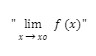This symbol indicates the limit value of a function. This process is generally used in geometry, hence, in mathematics, a limit is defined as the value that a function or sequence approaches as the index approaches a certain value.

The limit of a function is used in calculus in order to determine continuity, derivatives, and integrals. If you feel like practicing some limit-based exercises, you can find them online with given solutions so you can check your answers.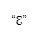You might recognize this symbol from Greek letters. The symbol for “epsilon” indicates a very small number close to 0.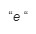This symbol marks the Euler’s number or the “E-constant/Euler’s constant”: e = 2.7182818284590452353602874713527… or shortly: “2.7 1828 1828”; where Euler’s number is an irrational number and it defines areas.

You might have guessed that the number is named after a great mathematician, Leonhard Euler who brought significant contributions to the number theory and calculus field.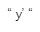The above symbol indicates a derivative in Lagrange’s notation considered the prime notation. A derivative number is the rate of change of a function in regard to one of its variables. Also referred to as differentiation.

Lagrange was a French mathematician concerned with celestial mechanics, besides number theory and analytical mathematics.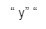It indicates the second derivative or derivative of a derivative in Lagrange’s notation.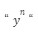Part of derivatives notation, this symbol indicates the “N times derivation”.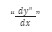In Leibniz’s notation, this fraction indicates a  derivative fraction. Leibniz was a German mathematician and philosopher influenced by great minds like Rene Descartes and Thomas Aquinas.

Leibniz’s notation is part of the derivatives that indicate change. This particular fraction denotes the fact that there are infinitely small (or infinitesimal) increments of x and y. It is considered a clearer indication of differentiation than prime notation.It indicates the derivative of a derivative; also if you substitute 2 with n, it becomes an “n-derivative” according to Leibniz’s notation. Mathematicians usually prefer Leibniz’s notation since it is more suitable for following complex functions.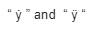According to Newton’s notation, this symbol indicates the time derivative. Used in physics and mechanics to solve velocity and acceleration problems, Newton’s notation is indicated by two dots above the letter.Notation in Euler’s mathematics, the first symbol indicating a derivative and the second symbol indicating a derivative of a derivative.This whole fraction indicates a partial derivative. Why do partial derivatives exist? In the case of multivariable derivatives, this fraction helps us see how the function changes when we change one variable while keeping the others constant.

### Integrals And Complex Numbers

“ ∫ “

This symbol indicates an integral number. An integral is either: a definite integral, which is the value equal to the area under the graph of a function for some interval, or, an indefinite integral which is a new function the derivative of which is the original function. There can be double or triple integrals, as well and they are indicated with the following symbol: “∬ “ double integral and “∭” for triple integral.

“∮”

This symbol indicates a closed contour or a line integral. In this type of geometrical operation, a contour offers a clear definition of curves on which an integral may be suitably defined.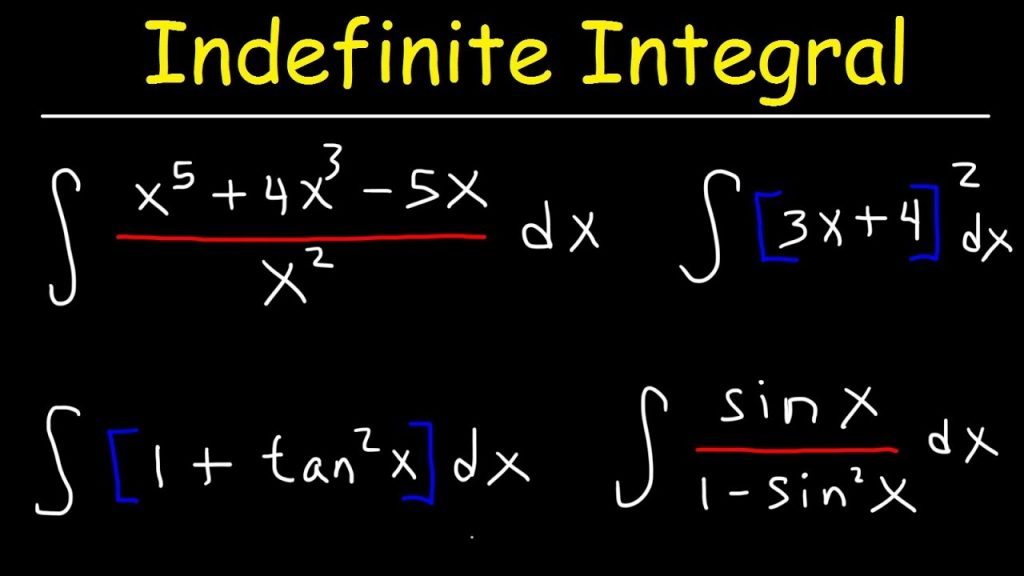### What Is Contour Integration?

Contour integration defines the process of calculating the value of a contour integral around a given contour in the complex plane. Please note the fact that these operators are used in complex geometrical mathematics and one might not encounter them in day-to-day mathematics.

“∯”

This symbol is used in order to indicate a closed surface integral, referring to the surface integrals of vector fields. Mathematics can get quite complicated at this point so if you are interested in vector fields, you can check more information on vector calculus online.

With a three closed integral symbol “∰ “ we indicate a closed volume integral. You can learn more about volumes and surfaces in calculus by looking at the Divergence Theorem

“ ∇ “ read as nabla or del, it is a divergence operator.

#### Other Symbols That You Might Encounter In Analytical Mathematics

• “ ⨯ “, also indicated with the implication → sign above x or the caret sign above x, it denotes the vector or a unit of a vector;

• “ x * y “ this pair indicates a convolution, which is the correlation function of f(τ) with the reversed function g(t-τ);

• “[a,b]”, indicates a closed interval;

• “ (a,b) “ this symbol stands for an open interval;

• “ i “ represents an imaginary unit;

• “ z* “ it is a complex conjugate, also indicated with a line above z, refers to the number with an equally real part and an imaginary part equal in magnitude but opposite in sign;

• “ Re(z) “ this symbol indicates the real part of a complex number

• “ Im(z) “ indicates the imaginary part of a complex number

• “ | z | “ indicates the absolute value/magnitude of a complex number

• “ arg(z) “is the argument of a complex number

• “ L “ this symbol indicates the Laplace transform, which is used to find solutions to differential equations and integrals.

• “ 𝐹 “ known as the Fourier transform refers to the mathematical technique that transforms a function of time, x(t), to a function of frequency, x(ω).

• “ ẟ “, the Delta function denotes a generalized function representing the limit of a class. Also known as the “Dirac’s delta function” or the “impulse symbol”;

• “ ∞ “

Possibly the most overused mathematical symbol, this sign stands for Lemniscate (any figure-eight or ∞-shaped curves) or more popularly known as the infinity symbol and it is used in algebraic geometry.

Don’t let these symbols scare you away from mathematical practice.

The Online Math Center provides easy-to-understand math tutoring programs for middle to high school students.

Reach out to us anytime and meet our math tutors! We are also here to help students that are interested in individual tutoring or competitions’ preparations.

## Step One

It only takes two steps to schedule a free lesson with an OMC representative.

To get started, please tell us who you are:

## Dear Parent!

Thank you for placing your trust in us to educate your child!

Congratulations on joining the Online Math Center!
See you in class.

## Thank you!

Our manager will be in contact with you shortly.

## Step Two

Let us know how to contact you. One of our representatives will get back to you shortly.

## Step Two

Awesome! We need to get in touch with your parent or guardian for further discussion. Please check in with them before filling out the form below with their information.

## Step One

It only takes two steps to schedule an appointment with an OMC representative.

To get started, please tell us who you are: